• Posts tagged "R"
• (Page 3)

# Blog Archives

## 信息熵的时间度量模型muti

R的极客理想系列文章，涵盖了R的思想，使用，工具，创新等的一系列要点，以我个人的学习和体验去诠释R的强大。

R语言作为统计学一门语言，一直在小众领域闪耀着光芒。直到大数据的爆发，R语言变成了一门炙手可热的数据分析的利器。随着越来越多的工程背景的人的加入，R语言的社区在迅速扩大成长。现在已不仅仅是统计领域，教育，银行，电商，互联网….都在使用R语言。

• 张丹(Conan), 程序员/Quant: Java,R,Nodejs
• blog: http://blog.fens.me
• email: bsspirit@gmail.com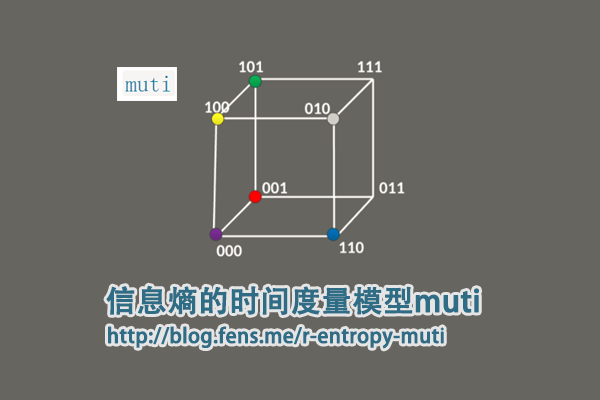1. muti包介绍
2. muti包函数定义
3. muti包使用
4. 实战案例

## 1. muti包介绍

muti包是用于计算两个离散随机变量之间的互信息(MI)的R包。 muti包设计目标，是考虑时间序列分析的情况下开发的，但没有任何方法可以将方法与时间索引本身联系起来。

muti项目的主页：https://mdscheuerell.github.io/muti/

muti包中用来计算互信息的计算过程，包括以下几步：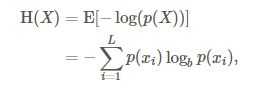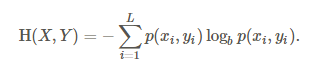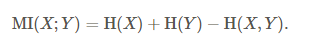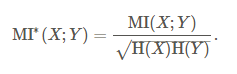• Win10 64bit
• R: 3.4.2 x86_64-w64-mingw32/x64 b4bit

muti目前还没有发布到CRAN上面，需要通过devtools包从github上进行安装。安装操作如下。

``````
> if(!require("devtools")) {
>  install.packages("devtools")
>  library("devtools")
> }
> devtools::install_github("mdscheuerell/muti")
``````

## 2. muti包函数定义

muti包，有很强的专业性，没有太多的函数定义，函数都是为了实现模型的算法。

• muti()，主函数，用于计算两个向量的互信息并画图输出
• mutual_info()，计算互信息
• newZ()，基于原始数据的重新采样创建新矢量。
• symbolize()，将数字向量转换为符号向量。
• transM()，估计用于计算互信息上的临界阈值的转换矩阵。
• plotMI()，画图输出

2.1 muti()函数：

``````
muti(x, y, sym = TRUE, n_bins = NULL, normal = FALSE, lags = seq(-4, 4), mc = 100, alpha = 0.05)
``````

• x, 向量
• y, 向量
• sym，是否转换成符号向量，默认TRUE,
• n_bins, 离散化方法，当sym=FALSE时， n_bins = ceiling（2 * length(x)^(1/3))
• normal, 是否标准化，默认FALSE
• lags, 滞后周期，默认为seq(-4, 4)
• mc, 用于估计互信息的临界阈值的蒙特卡洛数量的模拟，默认100,
• alpha, 用于定义临界阈值的取值点0.05

2.2 mutual_info()函数：

``````
# 源函数
mutual_info <- function(su,normal) {
## function to calculate mutual information between 2 time series
## need to remove obs with NA's b/c they don't contribute to info
su <- su[!apply(apply(su,1,is.na),2,any),]
## get number of bins
bb <- max(su)
## get ts length
TT <- dim(su)
## init entropies
H_s <- H_u <- H_su <- 0
for(i in 1:bb) {
## calculate entropy for 1st series
p_s <- sum(su[,1] == i)/TT
if(p_s != 0) { H_s <- H_s - p_s*log(p_s, 2) }
## calculate entropy for 2nd series
p_u <- sum(su[,2] == i)/TT
if(p_u != 0) { H_u <- H_u - p_u*log(p_u, 2) }
for(j in 1:bb) {
## calculate joint entropy
p_su <- sum(su[,1]==i & su[,2]==j)/TT
if(p_su != 0) { H_su <- H_su - p_su*log(p_su, 2) }
} ## end j loop
} ## end i loop
## calc mutual info
MI <- H_s + H_u - H_su
if(!normal) { return(MI) } else { return(MI/sqrt(H_s*H_u)) }
}

# 修改后的函数
library(philentropy)
mutual_info <- function(su,normal){
n<-nrow(su)
px<-table(su[,1])/n
py<-table(su[,2])/n

hX<-H(px)
hY<-H(py)

tf<-table(su[,1],su[,2])
pXY<-prop.table(tf)
hXY<-JE(pXY)

MI<-hX+hY-hXY
if(normal){
return(MI/sqrt(hX * hY))
}else{
return(MI)
}
}
``````

2.3 symbolize()函数：

``````
# 源函数
symbolize <- function(xy) {
## of interest and converts them from numeric to symbolic.
## check input for errors
if(dim(xy) != 2) {
stop("xy must be an [n x 2] matrix \n\n", call.=FALSE)
}
## get ts length
TT <- dim(xy)
## init su matrix
su <- matrix(NA, TT, 2)
## convert to symbols
## loop over 2 vars
for(i in 1:2) {
for(t in 2:(TT-1)) {
## if xy NA, also assign NA to su
if(any(is.na(xy[(t-1):(t+1),i]))) { su[t,i] <- NA }
## else get correct symbol
else {
if(xy[t,i] == xy[t-1,i] & xy[t,i] == xy[t+1,i]) {
## same
su[t,i] <- 3
}
if(xy[t,i] > xy[t-1,i]) {
## peak
if(xy[t,i] > xy[t+1,i]) { su[t,i] <- 5 }
## increase
else { su[t,i] <- 4 }
}
if(xy[t,i] < xy[t-1,i]) {
## trough
if(xy[t,i] < xy[t+1,i]) { su[t,i] <- 1 }
## decrease
else { su[t,i] <- 2 }
}
} ## end else
} ## end t loop
} ## end i loop
## return su matrix
return(su)
}

# 优化后的函数
symbolize<-function(xy){
if(ncol(xy)!=2) {
stop("xy must be an [n x 2] matrix \n\n", call. = FALSE)
}

idx<-2:(nrow(xy)-1)
apply(xy,2,function(row){
na<-which(is.na(row[idx]+row[idx-1]+row[idx+1]))
n3<-which(row[idx]==row[idx-1] & row[idx]==row[idx+1])
n5<-which(row[idx]>row[idx-1] & row[idx]>row[idx+1])
n4<-which(row[idx]>row[idx-1] & row[idx]<=row[idx+1])
n1<-which(row[idx]<row[idx-1] & row[idx]<row[idx+1])
n2<-which(row[idx]<row[idx-1] & row[idx]>=row[idx+1])

line<-rep(0,length(idx))
line[na]<-NA
line[n1]<-1
line[n2]<-2
line[n3]<-3
line[n4]<-4
line[n5]<-5
c(NA,line,NA)
})
}
``````

2.4 transM()函数：

``````
# 源函数
transM <- function(x,n_bins) {
## helper function for estimating transition matrix used in
## creating resampled ts for the CI on mutual info
## replace NA with runif()
x[is.na(x)] <- runif(length(x[is.na(x)]),min(x,na.rm=TRUE),max(x,na.rm=TRUE))
## length of ts
tt <- length(x)
## get bins via slightly extended range
bins <- seq(min(x)-0.001,max(x),length.out=n_bins+1)
## discretize ts
hin <- vector("numeric",tt)
for(b in 1:n_bins) {
hin[x > bins[b] & x <= bins[b+1]] <- b
}
## matrix of raw-x & discrete-x
xn <- cbind(x,hin)
## transition matrix from bin-i to bin-j
MM <- matrix(0,n_bins,n_bins)
for(i in 1:n_bins) {
for(j in 1:n_bins) {
MM[i,j] <- sum(hin[-tt]==i & hin[-1]==j)
}
if(sum(MM[i,])>0) { MM[i,] <- MM[i,]/sum(MM[i,]) }
else { MM[i,] <- 1/n_bins }
}
return(list(xn=xn,MM=MM))
} ## end function

# 优化后的函数
transM <- function(x,n_bins){
x[is.na(x)] <- runif(length(x[is.na(x)]), min(x, na.rm = TRUE),max(x, na.rm = TRUE))
n<-length(x)
xn<-cbind(x,hin=cut(x,7))

hin<-xn[,2]
a<-data.frame(id=1:(n-1),a=hin[1:(n-1)])
b<-data.frame(id=1:(n-1),b=hin[2:n])
ab<-merge(a,b,by="id",all=TRUE)
ab\$v<-1
abm<-aggregate(v~a+b,data=ab,sum)

MM <- matrix(0, n_bins, n_bins)
apply(abm,1,function(row){
MM[row,row]<<-row
invisible()
})

MN<-apply(MM,1,function(row){
s<-sum(row)
if(s>0){
row/s
}else{
rep(1/n_bins,n_bins)
}
})
MN<-t(MN)
return(list(xn = xn, MM = MN))
}
``````

## 3. muti包使用

3.1 数据的输入和输出
muti()函数，是muti包的主函数，调用muti()就完成了这个包的全部功能。

• 输入，要求是2个数字型向量。
• 输出，包括了2个部分，1.数据计算结果，2.可视化的结果。

``````> set.seed(123)
> TT <- 30

# 创建两个数字型向量
> x1 <- rnorm(TT)
> y1 <- x1 + rnorm(TT)

# 计算互信息
> muti(x1, y1)
lag MI_xy MI_tv
1  -4 0.238 0.595
2  -3 0.473 0.532
3  -2 0.420 0.577
4  -1 0.545 0.543
5   0 0.712 0.584
6   1 0.393 0.602
7   2 0.096 0.546
8   3 0.206 0.573
9   4 0.403 0.625
``````

• lag: 滞后期，当lag=-1，length(x)=length(y)=26时，x取值[1:25],y取值[2:26]；当lag=1，length(x)=length(y)=26时，x取值[2:26],y取值[1:25]；
• MI_xy: 互信息
• MI_tv: 相应显着性阈值，用蒙特卡洛方法生成互信息，按从大到小排序后取1-alpha位置的值。
• 上图，原始数据的x,y两个变量
• 中图，符号转型后的x,y两个变量，把原始数据离散化为5个值，差异化更大
• 下图，滞后期的互信息（实线）和相应显着性阈值（虚线），同数据计算结果的输出。

3.2 离散化处理

muti包提供了2种离散化。

• 符号化： 把连续型的数据，映射到5个值的上，分别对应peak(峰值), increasing(增加), same(没变)，decreasing(减少)，trough(低谷)。比如，向量c(1.1,2.1,3.3,1.2,3.1)，不计算第一个值和最后一个值，第二个值2.1>第一个值1.1，第二个值2.1<第三个值3.3时，第二个值为增加。第2，3，4值，分别为c(“增加”，“峰值”，“低谷”)。
• 分箱法： 等宽分箱，如果没有指定箱数，则根据莱斯规则计算，其中n = ceiling(2*length(x)^(1/3))。

## 4. 实战案例

``````// 省略部分代码
date X601398 X000001
1  2014-10-21    2.92 2339.66
2  2014-10-22    2.91 2326.55
3  2014-10-23    2.92 2302.42
4  2014-10-24    2.92 2302.28
5  2014-10-27    2.90 2290.44
6  2014-10-28    2.92 2337.87
7  2014-10-29    2.94 2373.03
8  2014-10-30    2.98 2391.08
9  2014-10-31    3.04 2420.18
10 2014-11-03    3.01 2430.03
11 2014-11-04    3.02 2430.68
12 2014-11-05    2.97 2419.25
13 2014-11-06    2.97 2425.86
14 2014-11-07    2.97 2418.17
15 2014-11-10    3.05 2473.67
16 2014-11-11    3.16 2469.67
17 2014-11-12    3.12 2494.48
18 2014-11-13    3.12 2485.61
19 2014-11-14    3.14 2478.82
20 2014-11-17    3.11 2474.01
``````

3列数据，第一列是时间列，第二列是X601398人收盘价，第三列是X000001收盘价。从价格上看，2个数据集并不在同一个度量内，我们转化度量单位为每日收益率。

``````> head(ret,20)
X601398       X000001
2014-10-21           NA            NA
2014-10-22 -0.003424658 -5.603378e-03
2014-10-23  0.003436426 -1.037158e-02
2014-10-24  0.000000000 -6.080559e-05
2014-10-27 -0.006849315 -5.142728e-03
2014-10-28  0.006896552  2.070781e-02
2014-10-29  0.006849315  1.503933e-02
2014-10-30  0.013605442  7.606309e-03
2014-10-31  0.020134228  1.217023e-02
2014-11-03 -0.009868421  4.069945e-03
2014-11-04  0.003322259  2.674864e-04
2014-11-05 -0.016556291 -4.702388e-03
2014-11-06  0.000000000  2.732252e-03
2014-11-07  0.000000000 -3.170010e-03
2014-11-10  0.026936027  2.295124e-02
2014-11-11  0.036065574 -1.617031e-03
2014-11-12 -0.012658228  1.004588e-02
2014-11-13  0.000000000 -3.555851e-03
2014-11-14  0.006410256 -2.731724e-03
2014-11-17 -0.009554140 -1.940439e-03
``````

``````> head(rdf,20)
X601398       X000001
2014-10-22 -0.003424658 -0.0056033783
2014-10-23  0.000000000 -0.0159168426
2014-10-24  0.000000000 -0.0159766804
2014-10-27 -0.006849315 -0.0210372447
2014-10-28  0.000000000 -0.0007650684
2014-10-29  0.006849315  0.0142627561
2014-10-30  0.020547945  0.0219775523
2014-10-31  0.041095890  0.0344152569
2014-11-03  0.030821918  0.0386252703
2014-11-04  0.034246575  0.0389030885
2014-11-05  0.017123288  0.0340177633
2014-11-06  0.017123288  0.0368429601
2014-11-07  0.017123288  0.0335561577
2014-11-10  0.044520548  0.0572775531
2014-11-11  0.082191781  0.0555679030
2014-11-12  0.068493151  0.0661720079
2014-11-13  0.068493151  0.0623808588
2014-11-14  0.075342466  0.0594787277
2014-11-17  0.065068493  0.0574228734
2014-11-18  0.058219178  0.0498833164
``````

``````
> x<-rdf\$X601398
> y<-rdf\$X000001
> result<-muti(x,y)
> result
lag MI_xy MI_tv
1  -4 0.148 0.534
2  -3 0.158 0.464
3  -2 0.190 0.385
4  -1 0.351 0.425
5   0 0.646 0.398
6   1 0.528 0.340
7   2 0.262 0.461
8   3 0.207 0.437
9   4 0.260 0.518
``````
• 上图，为原始数据的x(上证综指)和y(工商银行)的累积收益率曲线。
• 中图，为符号转型后的x(上证综指)和y(工商银行)的累积收益率曲线。
• 下图，符号转型后的数据，滞后期从-4到4的9个的互信息（实线）和和相应显着性阈值（虚线）。

``````// 省略部分代码
> rsdf
lag MI_xy MI_tv       sd
1  -4 0.148 0.534 1.991886
2  -3 0.158 0.464 2.400721
3  -2 0.190 0.385 2.107189
4  -1 0.351 0.425 2.378768
5   0 0.646 0.398 1.477030
6   1 0.528 0.340 2.342482
7   2 0.262 0.461 1.997676
8   3 0.207 0.437 2.267211
9   4 0.260 0.518 2.116934
``````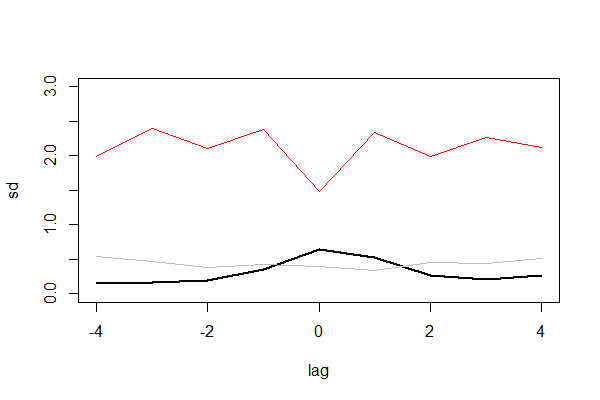## R语言统计特征描述包descriptr

R的极客理想系列文章，涵盖了R的思想，使用，工具，创新等的一系列要点，以我个人的学习和体验去诠释R的强大。

R语言作为统计学一门语言，一直在小众领域闪耀着光芒。直到大数据的爆发，R语言变成了一门炙手可热的数据分析的利器。随着越来越多的工程背景的人的加入，R语言的社区在迅速扩大成长。现在已不仅仅是统计领域，教育，银行，电商，互联网….都在使用R语言。

• 张丹(Conan), 程序员/Quant: Java,R,Nodejs
• blog: http://blog.fens.me
• email: bsspirit@gmail.com

http://blog.fens.me/r-descriptr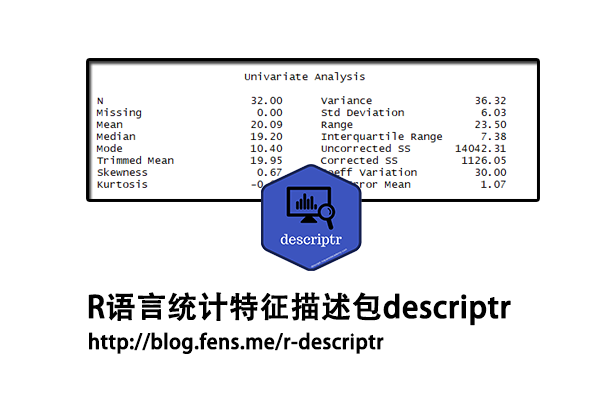descriptr包，为了我们提供了一套用来观察数据统计特征的工具集，主要特性包括统计特征计算，离散度，频率表，交叉表，分组摘要和多个单/双向表的度量，可以让我们非常方便的观察的数据特征。

1. descriptr包介绍
2. descriptr包函数列表
3. descriptr包函数使用

## 1. descriptr包介绍

descriptr包,主要用于生成描述性统计信息。它提供了3种数据处理视角，连续变量、类别变量（离散变量）和可视化。descriptr包的统计特征计算部分的源代码，结构非常工整，大量用到dplyr包来构建。

• Win10 64bit
• R: 3.4.2 x86_64-w64-mingw32/x64 b4bit

descriptr包的安装比较简单，直接用install.pacakges()函数就行。

``````
> install.packages("descriptr")
> library(descriptr)
``````

## 2. descriptr包函数列表

descriptr包，提供了3种处理视角，连续变量、类别变量、和可视化。我们将分别介绍这3种处理视角的函数。

descriptr包，提供了2个数据集，我们可以基于这2个数据集进行学习和测试。文中的例子，都是基于mtcarz的数据集进行构建的。

• hsb, 高中数据集
• mtcarz, 汽车数据集，复制系统的mtcars数据集

2.1 连续变量
2.1.1 统计概览

• ds_summary_stats, 统计概率
• ds_auto_summary_stats, 自动统计概率
• ds_group_summary, 分组描述性统计
• ds_auto_group_summary, 自动描述性统计
• ds_tidy_stats, 多变精简统计概率
• ds_multi_stats，已弃用函数，用ds_tidy_stats()替代

2.1.2 统计特征计算

• ds_mode, 计算众数
• ds_extreme_obs, 计算极端值
• ds_freq_cont, 计算频数
• ds_freq_table, 计算频率分布表
• ds_percentiles，计算分位数
• ds_range, 计算宽度, max(x)-min(x)
• ds_kurtosis, 计算峰度
• ds_skewness, 计算偏度
• ds_gmean, 计算几何平均值, prod(x)^(1/length(x))
• ds_hmean, 计算谐波均值, length(x)/sum(sapply(x, function(x) {1/x} ))
• ds_css, 计算修正平方和, sum((x1-mean)^2+(x2-mean)^2+…)
• ds_mdev, 计算平均绝对差, sum( abs(x1-mean) + abs(x2-mean) + …)
• ds_cvar, 计算变异系数, sd(x)/mean(x) * 100%
• ds_std_error, 计算标准误差, sd(x)/(length(x)^0.5)
• ds_tailobs，计算最大最小的多个值

2.1.3 度量特征

• ds_measures_location，位置的度量，包括均值，中位数和众数
• ds_measures_symmetry, 对称性的度量，包括峰度和偏度
• ds_measures_variation，变异的度量，包括宽度，方差，标准差

2.1.4 其他函数

• ds_rindex, 计算值的索引，同which
• ds_screener, 以表格展示数据

2.2 类别变量

• ds_twoway_table，计算双向表
• ds_cross_table, 展示双向表
• ds_auto_freq_table, 展示多个单向表
• ds_auto_cross_table, 展示多个双向表
• ds_tway_tables, 已弃用函数，用ds_auto_cross_table()替换
• ds_oway_tables，已弃用函数，用ds_auto_freq_table()替换

2.3 可视化
2.3.1 画图函数

• ds_plot_bar Generate bar plots
• ds_plot_bar_grouped Generate grouped bar plots
• ds_plot_bar_stacked Generate stacked bar plots
• ds_plot_box_group Compare distributions
• ds_plot_box_single Generate box plots
• ds_plot_density Generate density plots
• ds_plot_histogram Generate histograms
• ds_plot_scatter Generate scatter plots

2.3.2 已弃用函数，调用vistributions包

• dist_binom_perc, 可视化二项分布
• dist_binom_plot, 可视化二项分布
• dist_binom_prob，可视化二项分布
• dist_chi_perc, 可视化卡方分布
• dist_chi_plot, 可视化卡方分布
• dist_chi_prob, 可视化卡方分布
• dist_f_perc, 可视化F分布
• dist_f_plot, 可视化F分布
• dist_f_prob, 可视化F分布
• dist_norm_perc, 可视化正态分布
• dist_norm_plot, 可视化正态分布
• dist_norm_prob, 可视化正态分布
• dist_t_perc, 可视化T分布
• dist_t_plot, 可视化T分布
• dist_t_prob, 可视化T分布

2.4 演示小程序

• ds_launch_shiny_app, Shiny演示小程序

## 3. descriptr包函数使用

``````
> mtcarz
mpg cyl  disp  hp drat    wt  qsec vs am gear carb
Mazda RX4           21.0   6 160.0 110 3.90 2.620 16.46  0  1    4    4
Mazda RX4 Wag       21.0   6 160.0 110 3.90 2.875 17.02  0  1    4    4
Datsun 710          22.8   4 108.0  93 3.85 2.320 18.61  1  1    4    1
Hornet 4 Drive      21.4   6 258.0 110 3.08 3.215 19.44  1  0    3    1
Hornet Sportabout   18.7   8 360.0 175 3.15 3.440 17.02  0  0    3    2
Valiant             18.1   6 225.0 105 2.76 3.460 20.22  1  0    3    1
Duster 360          14.3   8 360.0 245 3.21 3.570 15.84  0  0    3    4
Merc 240D           24.4   4 146.7  62 3.69 3.190 20.00  1  0    4    2
Merc 230            22.8   4 140.8  95 3.92 3.150 22.90  1  0    4    2
Merc 280            19.2   6 167.6 123 3.92 3.440 18.30  1  0    4    4
Merc 280C           17.8   6 167.6 123 3.92 3.440 18.90  1  0    4    4
Merc 450SE          16.4   8 275.8 180 3.07 4.070 17.40  0  0    3    3
Merc 450SL          17.3   8 275.8 180 3.07 3.730 17.60  0  0    3    3
Merc 450SLC         15.2   8 275.8 180 3.07 3.780 18.00  0  0    3    3
Cadillac Fleetwood  10.4   8 472.0 205 2.93 5.250 17.98  0  0    3    4
Lincoln Continental 10.4   8 460.0 215 3.00 5.424 17.82  0  0    3    4
Chrysler Imperial   14.7   8 440.0 230 3.23 5.345 17.42  0  0    3    4
Fiat 128            32.4   4  78.7  66 4.08 2.200 19.47  1  1    4    1
Honda Civic         30.4   4  75.7  52 4.93 1.615 18.52  1  1    4    2
Toyota Corolla      33.9   4  71.1  65 4.22 1.835 19.90  1  1    4    1
Toyota Corona       21.5   4 120.1  97 3.70 2.465 20.01  1  0    3    1
Dodge Challenger    15.5   8 318.0 150 2.76 3.520 16.87  0  0    3    2
AMC Javelin         15.2   8 304.0 150 3.15 3.435 17.30  0  0    3    2
Camaro Z28          13.3   8 350.0 245 3.73 3.840 15.41  0  0    3    4
Pontiac Firebird    19.2   8 400.0 175 3.08 3.845 17.05  0  0    3    2
Fiat X1-9           27.3   4  79.0  66 4.08 1.935 18.90  1  1    4    1
Porsche 914-2       26.0   4 120.3  91 4.43 2.140 16.70  0  1    5    2
Lotus Europa        30.4   4  95.1 113 3.77 1.513 16.90  1  1    5    2
Ford Pantera L      15.8   8 351.0 264 4.22 3.170 14.50  0  1    5    4
Ferrari Dino        19.7   6 145.0 175 3.62 2.770 15.50  0  1    5    6
Maserati Bora       15.0   8 301.0 335 3.54 3.570 14.60  0  1    5    8
Volvo 142E          21.4   4 121.0 109 4.11 2.780 18.60  1  1    4    2
``````

3.1 数据展示

``````
# 查看数据静态结构
> ds_screener(mtcarz)
-----------------------------------------------------------------------
|  Column Name  |  Data Type  |  Levels   |  Missing  |  Missing (%)  |
-----------------------------------------------------------------------
|      mpg      |   numeric   |    NA     |     0     |       0       |
|      cyl      |   factor    |   4 6 8   |     0     |       0       |
|     disp      |   numeric   |    NA     |     0     |       0       |
|      hp       |   numeric   |    NA     |     0     |       0       |
|     drat      |   numeric   |    NA     |     0     |       0       |
|      wt       |   numeric   |    NA     |     0     |       0       |
|     qsec      |   numeric   |    NA     |     0     |       0       |
|      vs       |   factor    |    0 1    |     0     |       0       |
|      am       |   factor    |    0 1    |     0     |       0       |
|     gear      |   factor    |   3 4 5   |     0     |       0       |
|     carb      |   factor    |1 2 3 4 6 8|     0     |       0       |
-----------------------------------------------------------------------

Overall Missing Values           0
Percentage of Missing Values     0 %
Rows with Missing Values         0
Columns With Missing Values      0

# str()函数的静态结构
> str(mtcarz)
'data.frame':	32 obs. of  11 variables:
\$ mpg : num  21 21 22.8 21.4 18.7 18.1 14.3 24.4 22.8 19.2 ...
\$ cyl : Factor w/ 3 levels "4","6","8": 2 2 1 2 3 2 3 1 1 2 ...
\$ disp: num  160 160 108 258 360 ...
\$ hp  : num  110 110 93 110 175 105 245 62 95 123 ...
\$ drat: num  3.9 3.9 3.85 3.08 3.15 2.76 3.21 3.69 3.92 3.92 ...
\$ wt  : num  2.62 2.88 2.32 3.21 3.44 ...
\$ qsec: num  16.5 17 18.6 19.4 17 ...
\$ vs  : Factor w/ 2 levels "0","1": 1 1 2 2 1 2 1 2 2 2 ...
\$ am  : Factor w/ 2 levels "0","1": 2 2 2 1 1 1 1 1 1 1 ...
\$ gear: Factor w/ 3 levels "3","4","5": 2 2 2 1 1 1 1 2 2 2 ...
\$ carb: Factor w/ 6 levels "1","2","3","4",..: 4 4 1 1 2 1 4 2 2 4 ...
``````

3.2 统计概览

``````
# 统计概览
> ds_summary_stats(mtcarz,mpg)
-------------------------------------------- Variable: mpg --------------------------------------------

Univariate Analysis
N                       32.00      Variance                36.32
Missing                  0.00      Std Deviation            6.03
Mean                    20.09      Range                   23.50
Median                  19.20      Interquartile Range      7.38
Mode                    10.40      Uncorrected SS       14042.31
Trimmed Mean            19.95      Corrected SS          1126.05
Skewness                 0.67      Coeff Variation         30.00
Kurtosis                -0.02      Std Error Mean           1.07

Quantiles
Quantile                            Value
Max                                  33.90
99%                                  33.44
95%                                  31.30
90%                                  30.09
Q3                                   22.80
Median                               19.20
Q1                                   15.43
10%                                  14.34
5%                                   12.00
1%                                   10.40
Min                                  10.40

Extreme Values
Low                                High
Obs                        Value       Obs                        Value
15                         10.4        20                         33.9
16                         10.4        18                         32.4
24                         13.3        19                         30.4
7                         14.3        28                         30.4
17                         14.7        26                         27.3
``````

• Univariate Analysis（单变量分析），包括N（个数），Missing（缺失值），Mean（均值），Median（中位数），Mode（众数），Trimmed Mean（修正均值），Skewness（偏度），Kurtosis（峰度），Variance（方差），Std Deviation（标准差），Range(范围，最大-最小)，Interquartile Range(四分位数范围)，Uncorrected SS（未修正平方和），Corrected SS（修正平方和）， Coeff Variation（变异系数，标准差/均值），Std Error Mean（标准误差均值）
• Quantiles（分位数），从最小值到最小值，按顺序排列，对应的数值。
• Extreme Values（极值），包括最小值前5个，最大值前5个。

3.3 统计特征快速查看

``````
# 多变量统计
> ds_tidy_stats(mtcarz, mpg, disp, hp)
# A tibble: 3 x 16
vars    min   max  mean t_mean median  mode range variance  stdev  skew kurtosis coeff_var
<chr> <dbl> <dbl> <dbl>  <dbl>  <dbl> <dbl> <dbl>    <dbl>  <dbl> <dbl>    <dbl>     <dbl>
1 disp   71.1 472   231.   228    196.  276.  401.   15361.  124.   0.420  -1.07        53.7
2 hp     52   335   147.   144.   123   110   283     4701.   68.6  0.799   0.275       46.7
3 mpg    10.4  33.9  20.1   20.0   19.2  10.4  23.5     36.3   6.03 0.672  -0.0220      30.0
# ... with 3 more variables: q1 <dbl>, q3 <dbl>, iqrange <dbl>``````

3.4 频率表

``````
# 划分成5个等宽的频率
> ds_freq_table(mtcarz,mpg,5)
Variable: mpg
|-----------------------------------------------------------------------|
|    Bins     | Frequency | Cum Frequency |   Percent    | Cum Percent  |
|-----------------------------------------------------------------------|
| 10.4 - 15.1 |     6     |       6       |    18.75     |    18.75     |
|-----------------------------------------------------------------------|
| 15.1 - 19.8 |    12     |      18       |     37.5     |    56.25     |
|-----------------------------------------------------------------------|
| 19.8 - 24.5 |     8     |      26       |      25      |    81.25     |
|-----------------------------------------------------------------------|
| 24.5 - 29.2 |     2     |      28       |     6.25     |     87.5     |
|-----------------------------------------------------------------------|
| 29.2 - 33.9 |     4     |      32       |     12.5     |     100      |
|-----------------------------------------------------------------------|
|    Total    |    32     |       -       |    100.00    |      -       |
|-----------------------------------------------------------------------|
``````

3.5 分组统计

``````
> k<-ds_group_summary(mtcarz,cyl,mpg);k
mpg by cyl
-----------------------------------------------------------------------------------------
|     Statistic/Levels|                    4|                    6|                    8|
-----------------------------------------------------------------------------------------
|                  Obs|                   11|                    7|                   14|
|              Minimum|                 21.4|                 17.8|                 10.4|
|              Maximum|                 33.9|                 21.4|                 19.2|
|                 Mean|                26.66|                19.74|                 15.1|
|               Median|                   26|                 19.7|                 15.2|
|                 Mode|                 22.8|                   21|                 10.4|
|       Std. Deviation|                 4.51|                 1.45|                 2.56|
|             Variance|                20.34|                 2.11|                 6.55|
|             Skewness|                 0.35|                -0.26|                -0.46|
|             Kurtosis|                -1.43|                -1.83|                 0.33|
|       Uncorrected SS|              8023.83|              2741.14|              3277.34|
|         Corrected SS|               203.39|                12.68|                 85.2|
|      Coeff Variation|                16.91|                 7.36|                16.95|
|      Std. Error Mean|                 1.36|                 0.55|                 0.68|
|                Range|                 12.5|                  3.6|                  8.8|
|  Interquartile Range|                  7.6|                 2.35|                 1.85|
-----------------------------------------------------------------------------------------
``````

3.6 分组分类统计

``````
# 分组分类
> ds_auto_group_summary(mtcarz, cyl, gear, mpg)
mpg by cyl
-----------------------------------------------------------------------------------------
|     Statistic/Levels|                    4|                    6|                    8|
-----------------------------------------------------------------------------------------
|                  Obs|                   11|                    7|                   14|
|              Minimum|                 21.4|                 17.8|                 10.4|
|              Maximum|                 33.9|                 21.4|                 19.2|
|                 Mean|                26.66|                19.74|                 15.1|
|               Median|                   26|                 19.7|                 15.2|
|                 Mode|                 22.8|                   21|                 10.4|
|       Std. Deviation|                 4.51|                 1.45|                 2.56|
|             Variance|                20.34|                 2.11|                 6.55|
|             Skewness|                 0.35|                -0.26|                -0.46|
|             Kurtosis|                -1.43|                -1.83|                 0.33|
|       Uncorrected SS|              8023.83|              2741.14|              3277.34|
|         Corrected SS|               203.39|                12.68|                 85.2|
|      Coeff Variation|                16.91|                 7.36|                16.95|
|      Std. Error Mean|                 1.36|                 0.55|                 0.68|
|                Range|                 12.5|                  3.6|                  8.8|
|  Interquartile Range|                  7.6|                 2.35|                 1.85|
-----------------------------------------------------------------------------------------

mpg by gear
-----------------------------------------------------------------------------------------
|     Statistic/Levels|                    3|                    4|                    5|
-----------------------------------------------------------------------------------------
|                  Obs|                   15|                   12|                    5|
|              Minimum|                 10.4|                 17.8|                   15|
|              Maximum|                 21.5|                 33.9|                 30.4|
|                 Mean|                16.11|                24.53|                21.38|
|               Median|                 15.5|                 22.8|                 19.7|
|                 Mode|                 10.4|                   21|                   15|
|       Std. Deviation|                 3.37|                 5.28|                 6.66|
|             Variance|                11.37|                27.84|                44.34|
|             Skewness|                -0.09|                  0.7|                 0.56|
|             Kurtosis|                -0.38|                -0.77|                -1.83|
|       Uncorrected SS|              4050.52|               7528.9|              2462.89|
|         Corrected SS|               159.15|               306.29|               177.37|
|      Coeff Variation|                20.93|                21.51|                31.15|
|      Std. Error Mean|                 0.87|                 1.52|                 2.98|
|                Range|                 11.1|                 16.1|                 15.4|
|  Interquartile Range|                  3.9|                 7.08|                 10.2|
-----------------------------------------------------------------------------------------
``````

3.7 测量

``````
> ds_measures_variation(mtcarz)
# A tibble: 6 x 7
var    range     iqr  variance      sd coeff_var std_error
<chr>  <dbl>   <dbl>     <dbl>   <dbl>     <dbl>     <dbl>
1 disp  401.   205.    15361.    124.         53.7   21.9
2 drat    2.17   0.840     0.286   0.535      14.9    0.0945
3 hp    283     83.5    4701.     68.6        46.7   12.1
4 mpg    23.5    7.38     36.3     6.03       30.0    1.07
5 qsec    8.40   2.01      3.19    1.79       10.0    0.316
6 wt      3.91   1.03      0.957   0.978      30.4    0.173
``````

``````
> ds_measures_location(mtcarz)
# A tibble: 6 x 5
var     mean trim_mean median   mode
<chr>  <dbl>     <dbl>  <dbl>  <dbl>
1 disp  231.      228    196.   276.
2 drat    3.60      3.58   3.70   3.07
3 hp    147.      144.   123    110
4 mpg    20.1      20.0   19.2   10.4
5 qsec   17.8      17.8   17.7   17.0
6 wt      3.22      3.20   3.32   3.44
``````

``````
> ds_percentiles(mtcarz)
# A tibble: 6 x 12
var     min  per1  per5 per10     q1 median     q3  per95  per90  per99    max
<chr> <dbl> <dbl> <dbl> <dbl>  <dbl>  <dbl>  <dbl>  <dbl>  <dbl>  <dbl>  <dbl>
1 disp  71.1  72.5  77.4  80.6  121.   196.   326    449    396.   468.   472
2 drat   2.76  2.76  2.85  3.01   3.08   3.70   3.92   4.31   4.21   4.78   4.93
3 hp    52    55.1  63.6  66     96.5  123    180    254.   244.   313.   335
4 mpg   10.4  10.4  12.0  14.3   15.4   19.2   22.8   31.3   30.1   33.4   33.9
5 qsec  14.5  14.5  15.0  15.5   16.9   17.7   18.9   20.1   20.0   22.1   22.9
6 wt     1.51  1.54  1.74  1.96   2.58   3.32   3.61   5.29   4.05   5.40   5.42
``````

``````
> ds_extreme_obs(mtcarz,mpg)
# A tibble: 10 x 3
type  value index
<chr> <dbl> <int>
1 high   33.9    20
2 high   32.4    18
3 high   30.4    19
4 high   30.4    28
5 high   27.3    26
6 low    10.4    15
7 low    10.4    16
8 low    13.3    24
9 low    14.3     7
10 low    14.7    17
``````

3.8 类别变量频率表

``````
> ds_cross_table(mtcarz, cyl, gear)
Cell Contents
|---------------|
|     Frequency |
|       Percent |
|       Row Pct |
|       Col Pct |
|---------------|

Total Observations:  32

----------------------------------------------------------------------------
|              |                           gear                            |
----------------------------------------------------------------------------
|          cyl |            3 |            4 |            5 |    Row Total |
----------------------------------------------------------------------------
|            4 |            1 |            8 |            2 |           11 |
|              |        0.031 |         0.25 |        0.062 |              |
|              |         0.09 |         0.73 |         0.18 |         0.34 |
|              |         0.07 |         0.67 |          0.4 |              |
----------------------------------------------------------------------------
|            6 |            2 |            4 |            1 |            7 |
|              |        0.062 |        0.125 |        0.031 |              |
|              |         0.29 |         0.57 |         0.14 |         0.22 |
|              |         0.13 |         0.33 |          0.2 |              |
----------------------------------------------------------------------------
|            8 |           12 |            0 |            2 |           14 |
|              |        0.375 |            0 |        0.062 |              |
|              |         0.86 |            0 |         0.14 |         0.44 |
|              |          0.8 |            0 |          0.4 |              |
----------------------------------------------------------------------------
| Column Total |           15 |           12 |            5 |           32 |
|              |        0.468 |        0.375 |        0.155 |              |
----------------------------------------------------------------------------
``````

3.9 类别变量的双向表

``````
> ds_twoway_table(mtcarz, cyl, gear)
Joining, by = c("cyl", "gear", "count")
# A tibble: 8 x 6
cyl   gear  count percent row_percent col_percent
<fct> <fct> <int>   <dbl>       <dbl>       <dbl>
1 4     3         1  0.0312      0.0909      0.0667
2 4     4         8  0.25        0.727       0.667
3 4     5         2  0.0625      0.182       0.4
4 6     3         2  0.0625      0.286       0.133
5 6     4         4  0.125       0.571       0.333
6 6     5         1  0.0312      0.143       0.2
7 8     3        12  0.375       0.857       0.8
8 8     5         2  0.0625      0.143       0.4
``````

3.10 可视化连续型数据

``````
> ds_plot_histogram(mtcarz, mpg, disp)
> ds_plot_density(mtcarz, mpg, disp)
> ds_plot_box_single(mtcarz, mpg, disp)
> ds_plot_scatter(mtcarz, mpg, disp)
``````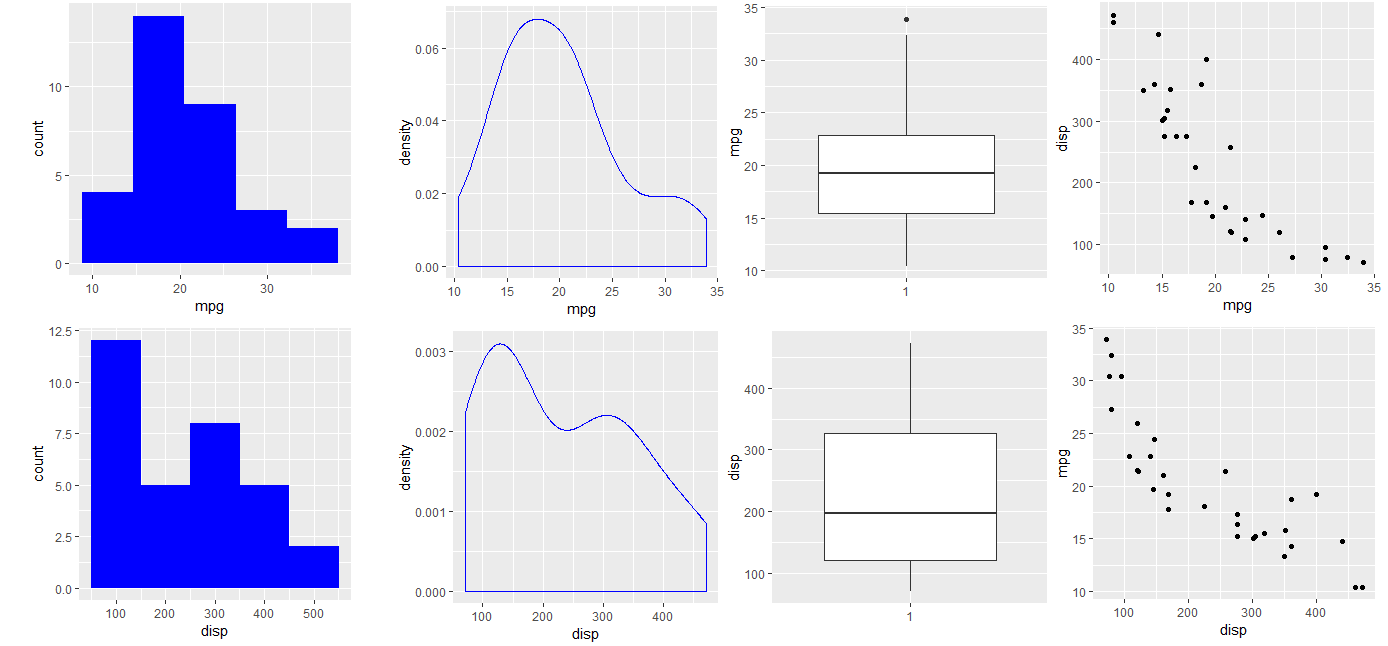3.11 可视化类别型数据

``````
> ds_plot_bar(mtcarz,cyl, gear)
> ds_plot_bar_stacked(mtcarz, cyl, gear)
> ds_plot_bar_grouped(mtcarz, cyl, gear)
> ds_plot_box_group(mtcarz, cyl, gear, mpg)
``````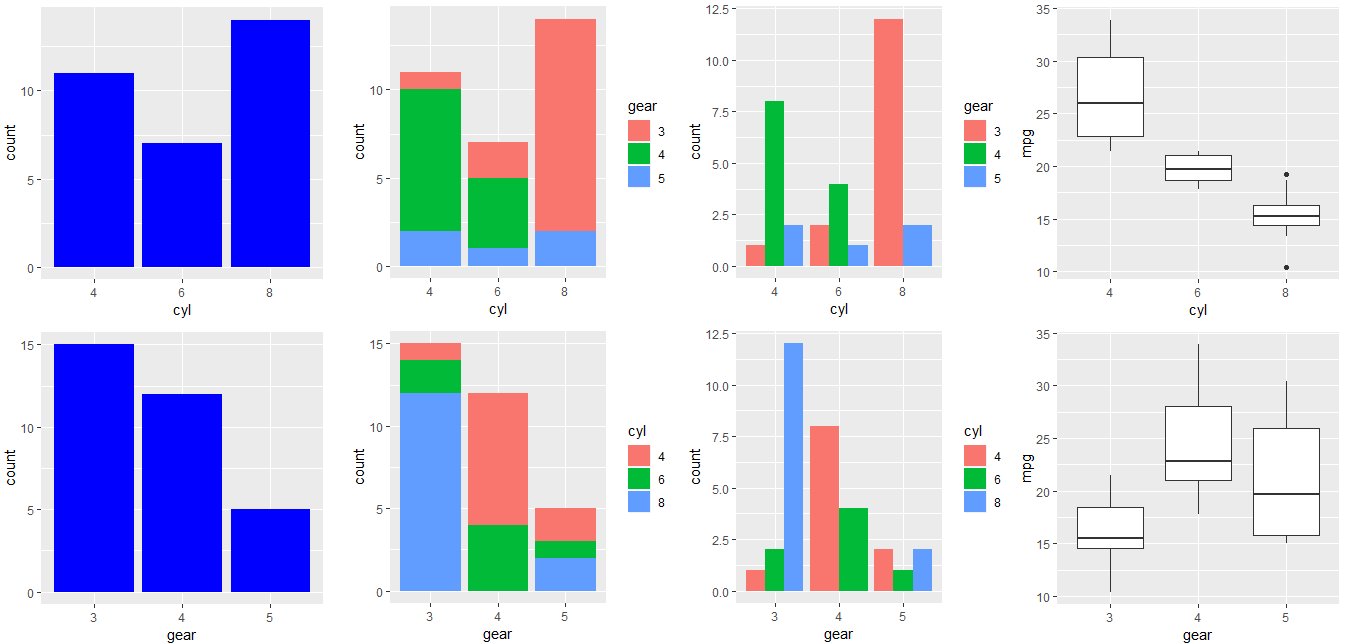3.12 可视化分布图

5种统计分布的可视化效果，由于使用时提示已弃用，改为调用vistributions包的对应函数，所以大家可以改用vistributions包。

``````
> dist_binom_prob(10, 0.3, 4, type = 'exact')
``````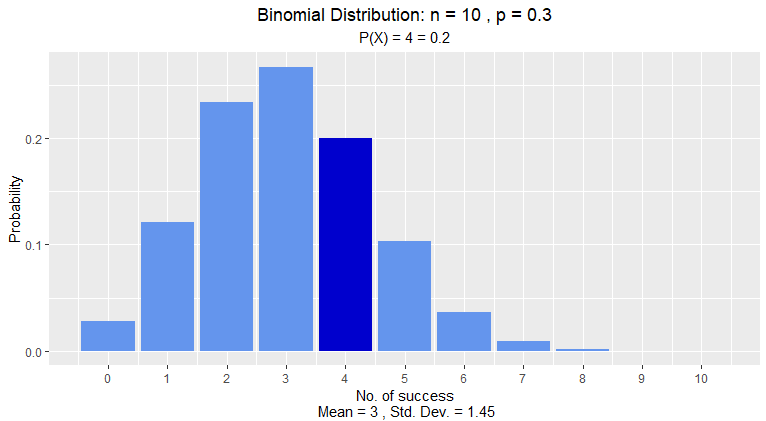``````
> dist_chi_perc(0.22, 13, 'upper')
``````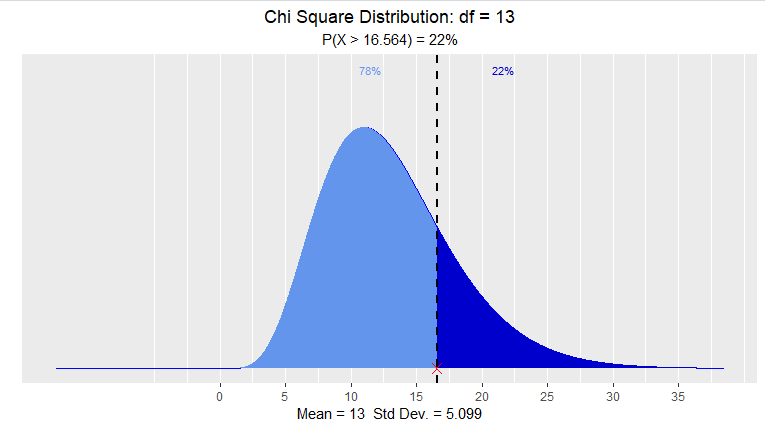F分布

``````
> dist_f_perc(0.125, 9, 35, 'upper')
``````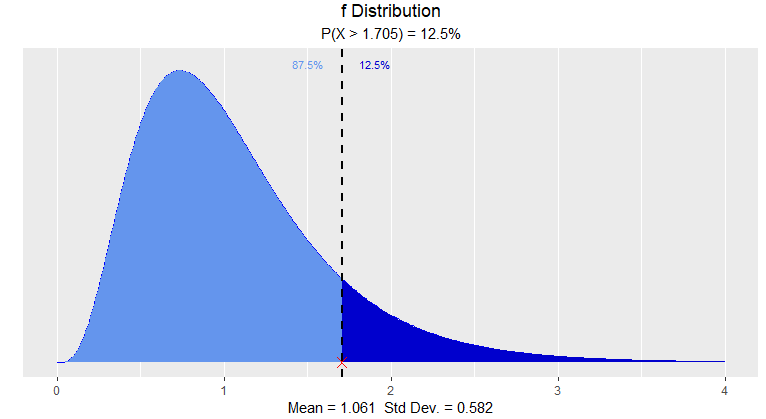``````
> dist_norm_perc(0.95, mean = 2, sd = 1.36, type = 'both')
``````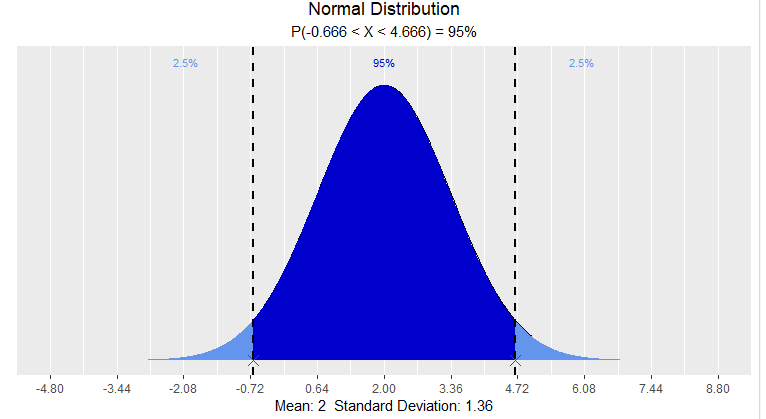T分布

``````
> dist_t_prob(1.445, 7, 'interval')
``````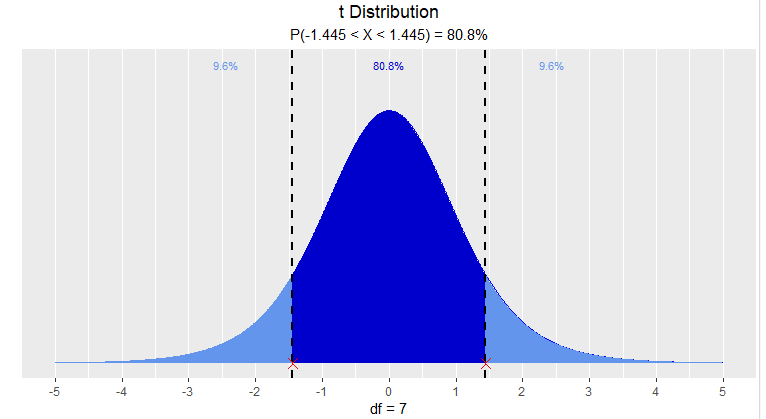3.13 启动shiny小程序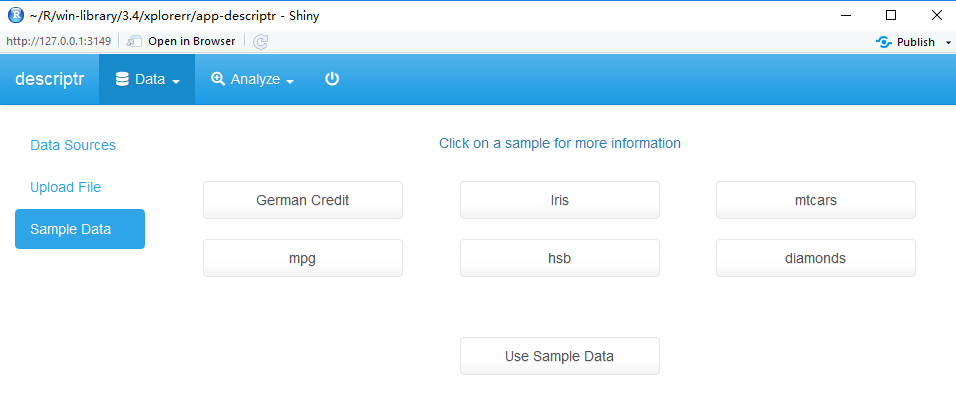http://blog.fens.me/r-descriptr## 2019中国R大会北京 : 凯利公式-用胜率和赔率量化你的投资

• 张丹(Conan), 程序员/Quant: Java,R,Nodejs
• blog: http://fens.me
• email: bsspirit@gmail.com1. 我分享的主题：凯利公式-用胜率和赔率量化你的投资
2. 会议体验和照片分享

## 1. 我分享的主题：凯利公式-用胜率和赔率量化你的投资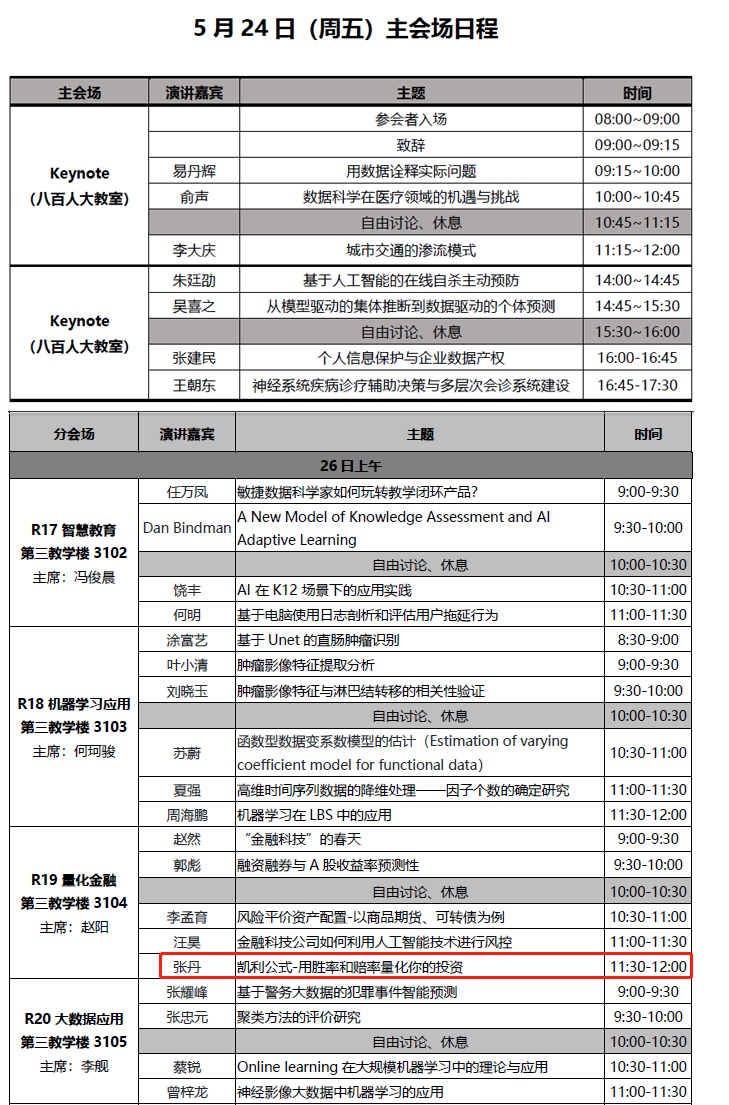• 故事开始：通过一个故事，带个场景。
• 凯利公式：原理，公式，变型。
• 赌局最优解：通过公式结合真实市场，定义不同场景下最优解。
• 让时间帮我们赚钱：用R语言程序，按假设条件进行仿真。

## 2. 会议体验和照片分享

2.1 会议体验证和总结

2.2 相关照片## R语言实现聚类kmeans

R的极客理想系列文章，涵盖了R的思想，使用，工具，创新等的一系列要点，以我个人的学习和体验去诠释R的强大。

R语言作为统计学一门语言，一直在小众领域闪耀着光芒。直到大数据的爆发，R语言变成了一门炙手可热的数据分析的利器。随着越来越多的工程背景的人的加入，R语言的社区在迅速扩大成长。现在已不仅仅是统计领域，教育，银行，电商，互联网….都在使用R语言。

• 张丹(Conan), 程序员/Quant: Java,R,Nodejs
• blog: http://blog.fens.me
• email: bsspirit@gmail.com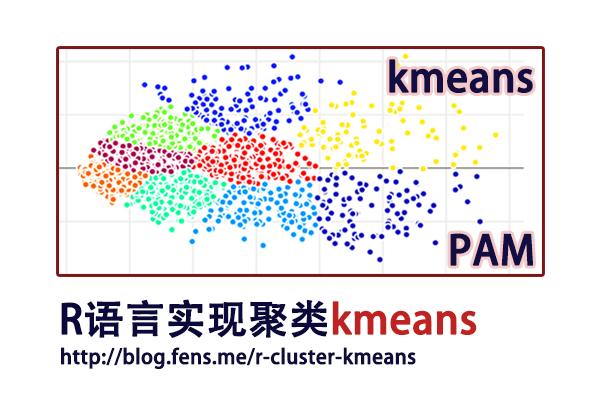1. k-means实现
2. PAM实现
3. 可视化和段剖面图

## 1. k-means实现

k-means算法，是一种最广泛使用的聚类算法。k-means以k作为参数，把数据分为k个组，通过迭代计算过程，将各个分组内的所有数据样本的均值作为该类的中心点，使得组内数据具有较高的相似度，而组间的相似度最低。

k-means工作原理：

1. 初始化数据，选择k个对象作为中心点。
2. 遍历整个数据集，计算每个点与每个中心点的距离，将它分配给距离中心最近的组。
3. 重新计算每个组的平均值，作为新的聚类中心。
4. 上面2-3步，过程不断重复，直到函数收敛，不再新的分组情况出现。

k-means聚类，适用于连续型数据集。在计算数据样本之间的距离时，通常使用欧式距离作为相似性度量。k-means支持多种距离计算，还包括maximum, manhattan, pearson, correlation, spearman, kendall等。各种的距离算法的介绍，请参考文章R语言实现46种距离算法

1.1 kmeans()函数实现

• Win10 64bit
• R: 3.4.4 x86_64-w64-mingw32

``````# 创建数据集
> set.seed(0)
> df <- rbind(matrix(rnorm(100, 0.5, 4.5), ncol = 2),
+             matrix(rnorm(100, 0.5, 0.1), ncol = 2))
> colnames(df) <- c("x", "y")
x          y
[1,]  6.1832943  1.6976181
[2,] -0.9680501 -1.1951622
[3,]  6.4840967 11.4861408
[4,]  6.2259319 -3.0790260
[5,]  2.3658865  0.2530514
[6,] -6.4297752  1.6256360
``````

``````
> cl <- kmeans(df,2); cl
K-means clustering with 2 clusters of sizes 14, 86

Cluster means:                   # 中心点坐标
x         y
1  5.821526 2.7343127
2 -0.315946 0.1992429

Clustering vector:               # 分组的索引
 1 2 1 1 2 2 2 2 2 1 1 2 2 2 2 2 2 2 2 2 2 2 2 1 2 1 1 2 2 2 2 2 2 2 2 1 1 2 1 2 1 2 2 2 2 2 2 1 1 2
 2 2 2 2 2 2 2 2 2 2 2 2 2 2 2 2 2 2 2 2 2 2 2 2 2 2 2 2 2 2 2 2 2 2 2 2 2 2 2 2 2 2 2 2 2 2 2 2 2 2

Within cluster sum of squares by cluster:
 316.0216 716.4009                       # withinss，分组内平方和
(between_SS / total_SS =  34.0 %)          # 组间的平方和/总平方和，用于衡量点聚集程度

Available components:            # 对象属性
 "cluster"      "centers"      "totss"        "withinss"     "tot.withinss" "betweenss"
 "size"         "iter"         "ifault"

# 查看数据分组情况，第1组86个，第2组14个
> cl\$size
 86 14
``````

• cluster，每个点的分组
• centers，聚类的中心点坐标
• totss，总平方和
• withinss，每个分组内的平方和
• tot.withinss，分组总和，sum(withinss)
• betweenss，组间的平方和，totss – tot.withinss
• size，每个组中的数据点数量
• iter，迭代次数。
• ifault，可能有问题的指标

1.2 kcca()函数实现

``````
# 安装flexclust包
> # install.packages("flexclust")
> library(flexclust)

# 进行聚类
> clk<-kcca(df,k=2);clk
kcca object of family ‘kmeans’

call:
kcca(x = df, k = 2)

cluster sizes:  # 聚类的分组大小
1  2
84 16

# 聚类的中心
> clk@centers
x         y
[1,] -0.3976465 0.2015319
[2,]  5.4832702 2.4054118

# 查看聚类的概览信息
> summary(clk)
kcca object of family ‘kmeans’

call:
kcca(x = df, k = 2)

cluster info:         # 每个组的基本信息，包括分组数量，平均距离、最大距离、分割值
size  av_dist max_dist separation
1   84 2.102458 9.748136   3.368939
2   16 3.972920 9.576635   3.189891

convergence after 5 iterations                   # 5次迭代
sum of within cluster distances: 240.1732        # 聚类距离之和
``````

``````> plot(df, col = cl\$cluster, main="Kmeans Cluster")
> points(cl\$centers, col = 1:3, pch = 10, cex = 4) # 画出kmeans()函数效果
``````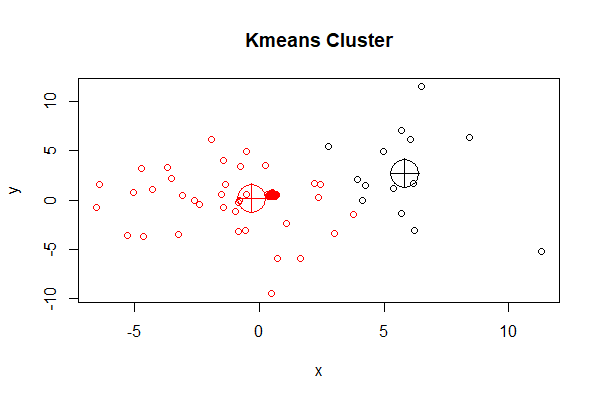``````> points(clk@centers, col = 3:4, pch = 10, cex = 4)  # 画出kcca()函数效果
``````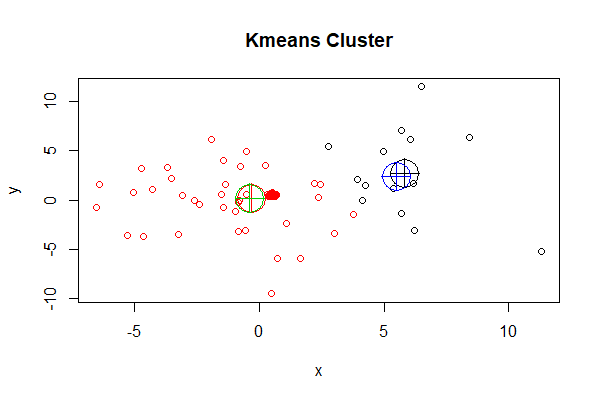k-means算法，也有一些缺点就是对于孤立点是敏感的，会被一些极端值影响聚类的效果。一种改进的算法是PAM，用于解决这个问题。PAM不使用分组平均值作为计算的参照点，而是直接使用每个组内最中心的对象作为中心点。

## 2. PAM实现

PAM(Partitioning Around Medoids)，又叫k-medoids，它可以将数据分组为k个组，k为数量是要事前定义的。PAM与k-means一样，找到距离中心点最小点组成同一类。PAM对噪声和异常值更具鲁棒性，该算法的目标是最小化对象与其最接近的所选对象的平均差异。PAM可以支持混合的数据类型，不仅限于连续变量。

PAM算法分为两个阶段：

1. 第1阶段BUILD，为初始集合S选择k个对象的集合。
2. 第2阶段SWAP，尝试用未选择的对象，交换选定的中心点，来提高聚类的质量。

PAM的工作原理：

1. 初始化数据集，选择k个对象作为中心。
2. 遍历数据点，把每个数据点关联到最近中心点m。
3. 随机选择一个非中心对象，与中心对象交换，计算交换后的距离成本
4. 如果总成本增加，则撤销交换的动作。
5. 上面2-4步，过程不断重复，直到函数收敛，中心不再改变为止。

• 消除了k-means算法对于孤立点的敏感性。
• 比k-means的计算的复杂度要高。
• 与k-means一样，必须设置k的值。
• 对小的数据集非常有效，对大数据集效率不高。

``````
> install.packages("cluster")
> library(cluster)
``````

pam()函数定义：

``````
pam(x, k, diss = inherits(x, "dist"), metric = "euclidean",
medoids = NULL, stand = FALSE, cluster.only = FALSE,
do.swap = TRUE,
keep.diss = !diss && !cluster.only && n < 100,
keep.data = !diss && !cluster.only,
pamonce = FALSE, trace.lev = 0)
``````

• x，数据框或矩阵，允许有空值(NA)
• k，设置分组数量
• diss，为TRUE时，x为距离矩阵；为FALSE时，x是变量矩阵。默认为FALSE
• metric，设置距离算法，默认为euclidean，距离矩阵忽略此项
• medoids，指定初始的中心，默认为不指定。
• stand，为TRUE时进行标准化，距离矩阵忽略此项。
• cluster.only，为TRUE时，仅计算聚类结果，默认为FALSE
• do.swap，是否进行中心点交换，默认为TRUE；对于超大的数据集，可以不进行交换。
• keep.diss，是否保存距离矩阵数据
• keep.data，是否保存原始数据
• pamonce，一种加速算法，接受值为TRUE,FALSE,0,1,2
• trace.lev，日志打印，默认为0，不打印

``````> kclus <- pam(df,2)

# 查看kclus对象
> kclus
Medoids:                                     # 中心点
ID         x         y
[1,] 27 5.3859621 1.1469717
[2,] 89 0.4130217 0.4798659

Clustering vector:                           # 分组
 1 2 1 1 2 2 2 2 2 1 1 2 2 2 2 2 2 2 2 2 2 2 2 1 2 1 1 2 2 2 2 2 2 2 1 1 1 2 1 2 1 2 2 2 2 2 2 1 1 2
 2 2 2 2 2 2 2 2 2 2 2 2 2 2 2 2 2 2 2 2 2 2 2 2 2 2 2 2 2 2 2 2 2 2 2 2 2 2 2 2 2 2 2 2 2 2 2 2 2 2

Objective function:                          # 目标函数的局部最小值
build     swap
2.126918 2.124185

Available components:                        # 聚类对象的属性
 "medoids"    "id.med"     "clustering" "objective"  "isolation"  "clusinfo"   "silinfo"
 "diss"       "call"       "data"

> kclus\$clusinfo        # 聚类的分组数量，每个组的平均距离、最大距离、分割值
size  max_diss  av_diss diameter separation
[1,]   15 10.397323 4.033095 17.35984   1.556862
[2,]   85  9.987604 1.787318 15.83646   1.556862
``````

• medoids，中心点的数据值
• id.med，中心点的索引
• clustering，每个点的分组
• objective，目标函数的局部最小值
• isolation，孤立的聚类(用L或L*表示)
• clusinfo，每个组的基本信息
• silinfo，存储各观测所属的类、其邻居类以及轮宽（silhouette）值
• diss，不相似度
• call，执行函数和参数
• data，原始数据集

``````# 画图
> plot(df, col = kclus\$clustering, main="Kmedoids Cluster")
> points(kclus\$medoids, col = 1:3, pch = 10, cex = 4)
``````

##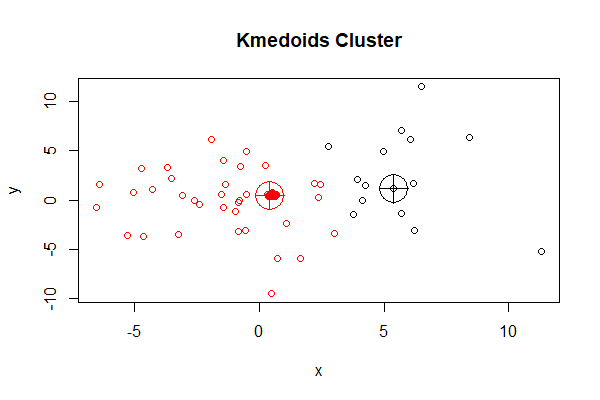``````
# 设置聚类的中心为1，2
> kclus2<-pam(df,2,medoids=c(1,2),trace.lev=20)
C pam(): computing 4951 dissimilarities from  100 x 2  matrix: [Ok]
pam()'s bswap(*, s=21.837, pamonce=0): medoids given
after build: medoids are   1   2
and min.dist dysma[1:n] are
0      0   9.79   4.78   3.63   6.15   5.23  0.929   8.44   8.59
2.29   2.69   4.48   1.19   1.98   2.81   5.39    4.2   3.72   4.56
1.84   3.99    2.4    2.7   4.84   5.08  0.969   2.01   4.94   5.06
1.94    7.4   5.19   1.62   3.94   3.12   3.51   0.65   4.46   4.61
5.16   4.57   1.82   3.21   5.79   4.01   5.59   5.38   1.95    6.2
2.41   2.09    2.2   2.43   2.24   2.26   2.09   2.39   2.21   2.33
2.24   2.14   2.45   2.37    2.2   2.37   2.13   2.33   2.25   2.18
2.38   2.19   2.15   2.14    2.1   2.39   2.24   2.24   2.12   2.14
2.34   2.18   2.25   2.26   2.33   2.17   2.18   2.12   2.17   2.27
2.29   2.26   2.38   2.12   2.25   2.33   2.09   2.21   2.24   2.13
swp new  89 <->   2 old; decreasing diss. 306.742 by -93.214
swp new  27 <->   1 old; decreasing diss. 213.528 by -1.10916
end{bswap()}, end{cstat()}

# 查看中心
> kclus2\$id.med
 27 89
``````

PAM作为k-means的一种改进算法，到底结果是否更合理，还要看最终哪种结果能够准确地表达业务的含义，被业务人员所认可，就需要不断地和业务人员来沟通。

## 3. 可视化和段剖面图

``````> res <- kmeans(iris[,1:4], centers=3)
> pairs(iris, col = res\$cluster + 1)
``````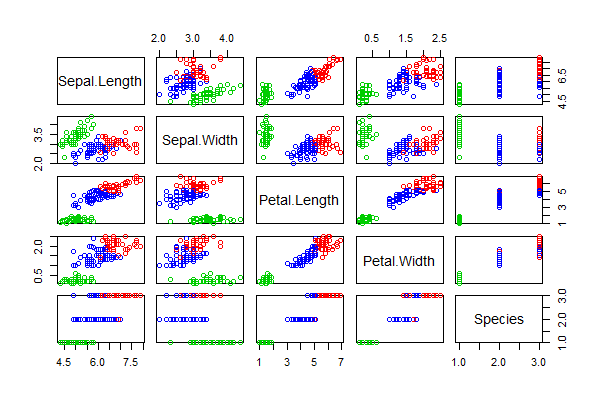``````> library(GGally)
> ggpairs(iris,columns = 1:5,mapping=aes(colour=as.character(res\$cluster)))
``````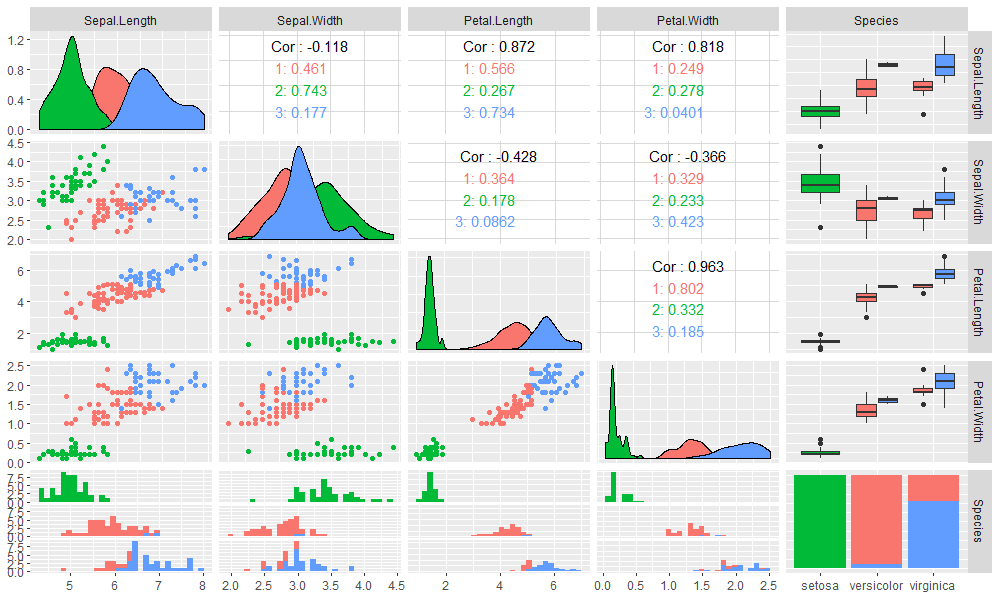``````
> library(flexclust)
> clk2 <- cclust(iris[,-5], k=3);clk2
kcca object of family ‘kmeans’

call:
cclust(x = iris[, -5], k = 3)

cluster sizes:
1  2  3
39 61 50

# 画出段剖面图
> barchart(clk2,legend=TRUE)
``````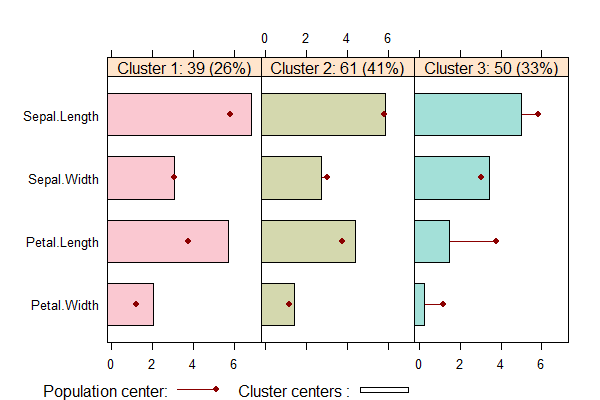• Cluster1中，有39个数据点占26%，Sepal.Width指标在均值附近，其他指标都大于均值。
• Cluster2中，有61个数据点占41%，Sepal.Width指标略小于均值，其他指标在均值附近。
• Cluster3中，有50个数据点占33%，Sepal.Width略大于均值，其他指标都小于均值。## 用R语言实现信息度量

R的极客理想系列文章，涵盖了R的思想，使用，工具，创新等的一系列要点，以我个人的学习和体验去诠释R的强大。

R语言作为统计学一门语言，一直在小众领域闪耀着光芒。直到大数据的爆发，R语言变成了一门炙手可热的数据分析的利器。随着越来越多的工程背景的人的加入，R语言的社区在迅速扩大成长。现在已不仅仅是统计领域，教育，银行，电商，互联网….都在使用R语言。

• 张丹(Conan), 程序员/Quant: Java,R,Nodejs
• blog: http://blog.fens.me
• email: bsspirit@gmail.com

http://blog.fens.me/r-entropy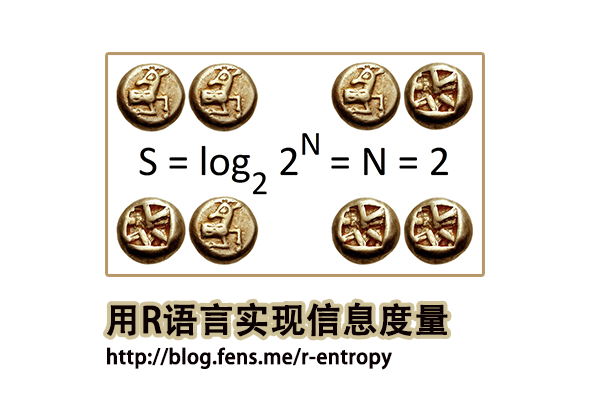1. 信息熵介绍
2. 关键概念
3. 信息度量函数
4. 应用举例

## 1.信息熵介绍

``````H(A) = -1*(0.2*log2(0.2)*5)
= 2.321928 bits``````

``````
H(B)=-1*(0.1*log2(0.1)+0.2*log2(0.2)+0.45*log2(0.45)+0.15*log2(0.15)+0.1*log2(0.1))
= 2.057717 bits
``````

## 2. 关键概念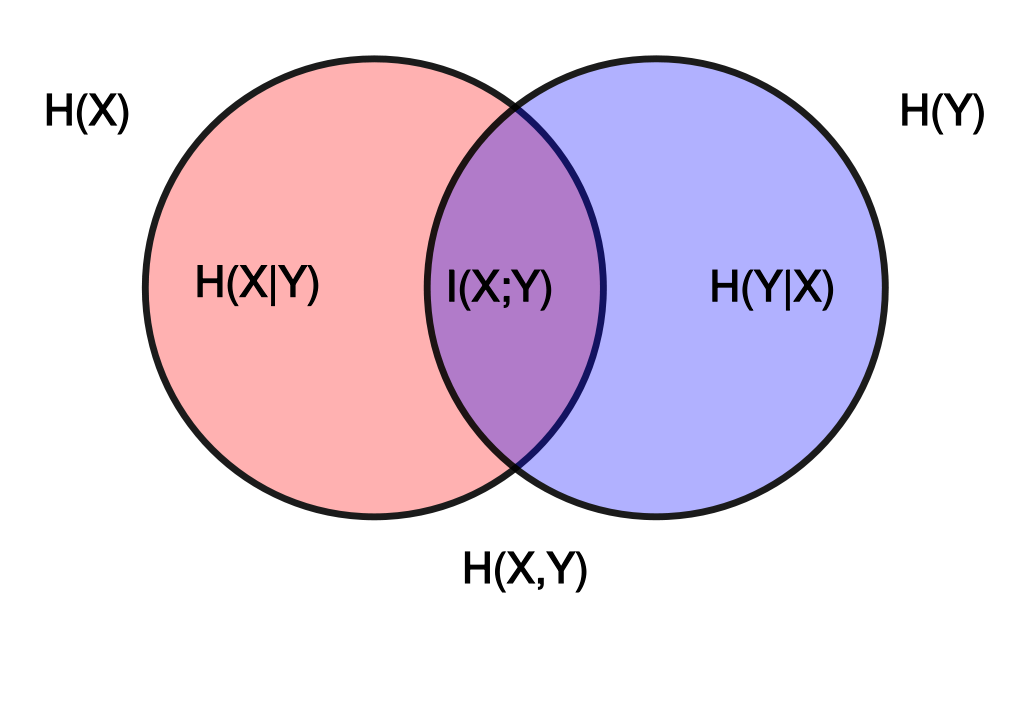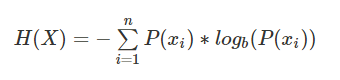H(x)的取值范围，0<=H(x)<=log(n), 其中n是随机变量x取值的种类数。需要注意的是，熵只依赖于随机变量的分布，与随机变量取值无关。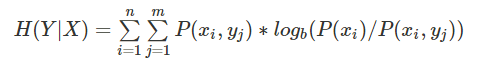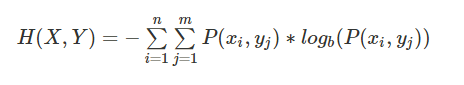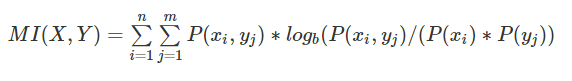KL散度（Kullback–Leibler Divergence, 相对熵）：随机变量x取值的两个概率分布p和q，用来衡量这2个分布的差异，通常用p表示真实分布，用q表示预测分布。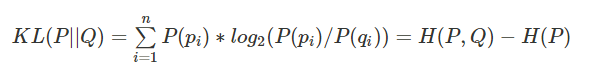n为事件的所有可能性，如果两个分布完全相同,那么它们的相关熵为0。如果相对熵KL越大，说明它们之间的差异越大，反之相对熵KL越小，说明它们之间的差异越小。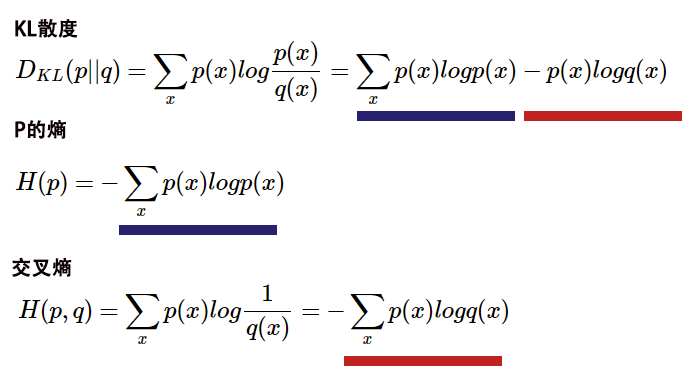## 3. 信息度量函数

philentropy包的函数，主要分为3种类别的函数，第一类是距离测量的函数，第二类是相关性分析，第三类是信息度量函数，本文重点介绍这些信息度量的函数。有关于距离测量函数和相关性分析函数，请参考文章R语言实现46种距离算法

• H(): 香农熵, Shannon’s Entropy H(X)
• JE() : 联合熵, Joint-Entropy H(X,Y)
• CE() : 条件熵, Conditional-Entropy H(X|Y)
• MI() : 互信息, Shannon’s Mutual Information I(X,Y)
• KL() : KL散度, Kullback–Leibler Divergence
• JSD() : JS散度，Jensen-Shannon Divergence
• gJSD() : 通用JS散度，Generalized Jensen-Shannon Divergence

• Win10 64bit
• R: 3.4.2 x86_64-w64-mingw32

3.1 H()香农熵
H()函数，可用于快速计算任何给定概率向量的香农熵。

H()函数定义：

``````H (x, unit = "log2")
``````

• x, 概率向量
• unit，对数化的单位，默认为log2

``````
# 创建数据x
> x<-1:10;x
  1  2  3  4  5  6  7  8  9 10
> px<-x/sum(x);x1
 0.01818182 0.03636364 0.05454545 0.07272727
 0.09090909 0.10909091 0.12727273 0.14545455
 0.16363636 0.18181818

# 计算香农熵
> H(px)
 3.103643
``````

``````
# 创建数据x
> x<-1:10
#计算x的概率密度px
> px<-x/sum(x)

# 根据公式计算香农熵
> -1*sum(px*log2(px))
 3.103643
``````

3.2 CE()条件熵

CE()函数，基于给定的联合概率向量P(X,Y)和概率向量P(Y)，根据公式 H(X|Y)= H(X,Y)-H(Y)计算香农的条件熵。

``CE(xy, y, unit = "log2")``

• xy, 联合概率向量
• y, 概率向量，必须是随机变量y的概率分布
• unit，对数化的单位，默认为log2

``````
> x3<- 1:10/sum(1:10)
> y3<- 30:40/sum(30:40)

# 计算条件熵
> CE(x3, y3)
 -0.3498852
``````

3.3 JE()联合熵

JE()函数，基于给定的联合概率向量P(X,Y)计算香农的联合熵H(X,Y)。

JE()函数定义：

``````JE (x, unit = "log2")
``````

• x, 联合概率向量
• unit，对数化的单位，默认为log2

``````# 创建数据x
> x2 <- 1:100/sum(1:100)

# 联合熵
> JE(x2)
 6.372236
``````

3.4 MI()互信息
MI()函数，根据给定联合概率向量P(X,Y)、概率向量P(X)和概率向量P(X)，按公式I(X,Y)= H(X)+ H(Y)-H(X,Y)计算。

``MI(x, y, xy, unit = "log2")``

• x, 概率向量
• x, 概率向量
• xy, 联合概率向量
• unit，对数化的单位，默认为log2

``````
# 创建数据集
> x3 <- 1:10/sum(1:10)
> y3<- 20:29/sum(20:29)
> xy3 <- 1:10/sum(1:10)

# 计算互信息
> MI(x3, y3, xy3)
 3.311973
``````

3.5 KL()散度
KL()函数，计算两个概率分布P和Q的Kullback-Leibler散度。

``KL(x, test.na = TRUE, unit = "log2", est.prob = NULL)``

• x, 概率向量或数据框
• test.na, 是否检查NA值
• unit，对数化的单位，默认为log2
• est.prob, 用计数向量估计概率的方法，默认值NULL。

``````
# 创建数据集
> df4 <- rbind(x3,y3);df4
[,1]       [,2]       [,3]       [,4]       [,5]      [,6]      [,7]      [,8]      [,9]
x3 0.01818182 0.03636364 0.05454545 0.07272727 0.09090909 0.1090909 0.1272727 0.1454545 0.1636364
y3 0.08163265 0.08571429 0.08979592 0.09387755 0.09795918 0.1020408 0.1061224 0.1102041 0.1142857
[,10]
x3 0.1818182
y3 0.1183673

# 计算KL散度
> KL(df4, unit = "log2") # Default
kullback-leibler
0.1392629
> KL(df4, unit = "log10")
kullback-leibler
0.0419223
> KL(df4, unit = "log")
kullback-leibler
0.09652967
``````

3.5 JSD()散度

JSD()函数，基于具有相等权重的Jensen-Shannon散度，计算距离矩阵或距离值。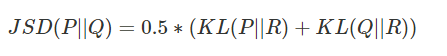``JSD(x, test.na = TRUE, unit = "log2", est.prob = NULL)``

• x, 概率向量或数据框
• test.na, 是否检查NA值
• unit, 对数化的单位，默认为log2
• est.prob, 用计数向量估计概率的方法，默认值NULL。
``````
# 创建数据
> x5 <- 1:10
> y5 <- 20:29
> df5 <- rbind(x5,y5)

# 计算JSD
> JSD(df5,unit='log2')
jensen-shannon
50.11323
> JSD(df5,unit='log')
jensen-shannon
34.73585
> JSD(df5,unit='log10')
jensen-shannon
15.08559

# 计算JSD，满足est.prob
> JSD(df5, est.prob = "empirical")
jensen-shannon
0.03792749
``````

3.6 gJSD()散度

gJSD()函数，计算概率矩阵的广义Jensen-Shannon散度。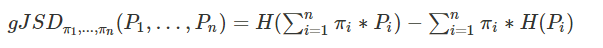``gJSD(x, unit = "log2", weights = NULL)``

• x, 概率矩阵
• unit, 对数化的单位，默认为log2
• weights, 指定x中每个值的权重，默认值NULL。
``````
# 创建数据
> Prob <- rbind(1:10/sum(1:10), 20:29/sum(20:29), 30:39/sum(30:39))

# 计算gJSD
> gJSD(Prob)
 0.023325
``````

## 4. 应用举例

``````
X,Y
Tourism,Y
Food,N
IT,Y
Tourism,N
Tourism,N
IT,Y
Food,N
Tourism,Y
``````

 X Y N p(X) Tourism 2/8=0.25 2/8=0.25 0.25+0.25=0.5 Food 0/8=0 2/8=0.25 0+0.25=0.25 IT 2/8=0.25 0/8=0 0.25+0=0.25 p(Y) 0.25+0+0.25=0.5 0.25+0.25+0=0.5

``````
# 分别计算每种情况的概率
p(X=Tourism) = 2/8 + 2/8 = 0.5
p(X=Food) = 2/8 + 0/8 = 0.25
p(X=IT) = 0/8 + 2/8 = 0.25
p(Y=Y) = 4/8 = 0.5
p(Y=N) = 4/8 = 0.5

# 计算H(X)
H(X) = -∑p(xi)*log2(p(xi))
= -p(X=Tourism)*log2(p(X=Tourism) ) -p(X=Food)*log2(p(X=Food) ) -p(X=IT)*log2(p(X=IT) )
= -0.5*log(0.5) -0.25*log(0.25) - 0.25*log(0.25)
= 1.5

# 计算H(Y)
H(Y) = -∑p(yi)*log2(p(yi))
= -p(Y=Y)*log2(p(Y=Y)) -p(Y=N)*log2(p(Y=N))
= -0.5*log(0.5) -0.5*log(0.5)
= 1
``````

``````
H(Y|X=Tourism) = -p(Y|X=Tourism)*log(p(Y|X=Tourism)) - p(N|X=Tourism)*log(p(N|X=Tourism))
= -0.5*log(0.5) -0.5*log(0.5)
= 1

H(Y|X=Food) = -p(Y|X=Food)*log(p(Y|X=Food)) -p(N|X=Food)*log(p(N|X=Food))
= -0*log(0) -1*log(1)
= 0

H(Y|X=IT) = -p(Y|X=IT)*log(p(Y|X=IT)) -p(N|X=IT)*log(p(N|X=IT))
= -1*log(1) -0*log(0)
= 0
``````

``````
H(Y|X) = ∑p(xi)*H(Y|xi)
= p(X=Tourism)*H(Y|X=Tourism) + p(X=Food)*H(Y|X=Food) + p(X=IT)*H(Y|X=IT)
= 0.5*1 + 0.25*0 + 0.25*0
= 0.5
``````

``````
H(X,Y) = −∑p(x,y)log(p(x,y))
= H(X) + H(Y|X)
= 1.5 + 0.5
= 2
``````

``````
I(X;Y) = H(Y) - H(Y|X)  = 1 - 0.5 = 0.5
= H(X) + H(Y) - H(X,Y) = 1.5 + 1 - 2 = 0.5
``````

``````
# 创建数据集
> X<-c('Tourism','Food','IT','Tourism','Tourism','IT','Food','Tourism')
> Y<-c('Y','N','Y','N','N','Y','N','Y')
> df<-cbind(X,Y);df
X         Y
[1,] "Tourism" "Y"
[2,] "Food"    "N"
[3,] "IT"      "Y"
[4,] "Tourism" "N"
[5,] "Tourism" "N"
[6,] "IT"      "Y"
[7,] "Food"    "N"
[8,] "Tourism" "Y
``````

``````
> tf<-table(df[,1],df[,2]);tf

N Y
Food    2 0
IT      0 2
Tourism 2 2
``````

``````
> pX<-margin.table(tf,1)/margin.table(tf);pX
Tourism    Food      IT
0.50    0.25    0.25
> pY<-margin.table(tf,2)/margin.table(tf);pY
Y   N
0.5 0.5
> pXY<-prop.table(tf);pXY
Y    N
Tourism 0.25 0.25
Food    0.00 0.25
IT      0.25 0.00
``````

``````
> H(pX)
 1.5
> H(pY)
 1

# 条件熵
> CE(pX,pY)
 0.5

# 联合熵
> JE(pXY)
 2

# 互信息
> MI(pX,pY,pXY)
 0.5
``````

http://blog.fens.me/r-entropy